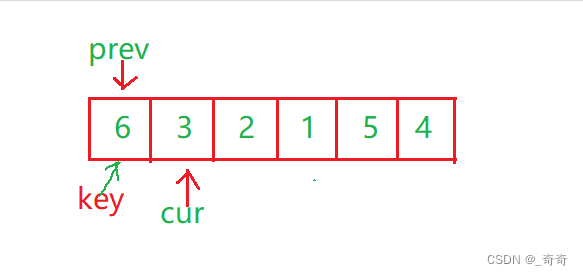# C語言植物大戰數據結構快速排序圖文示例

“田傢少閑月，五月人倍忙”“夜來南風起，小麥覆隴黃”

## 一、經典1962年Hoare法

```void QuickSort(int* a, int left, int right)
{
//請你完善以下代碼
}
int main()
{
int arr[] = {6,1,2,7,9,3,4,5,10,8};
int sz = sizeof(arr) / sizeof(arr);
QuickSort(arr, 0, sz-1);
return 0;
}
```

Hoare法和二叉樹的**前序遍歷(根 左子樹 右子樹)**簡直一模一樣。

1.先進行一趟排序。

2.進行左半區間(左子樹)遞歸。

3.進行右半區間(右子樹)遞歸。

### 1.單趟排序1.設置一個基準值key，key一般為左邊第一個數的下標。定義左指針left和有指針right分別指向第一個和最後一個。

2.先讓右邊的right指針往左走，一直找比key所指向小的數，找到後就停下。

3.緊接著讓left指針往右走，一直找比key所指向大的數，找到後就停下。

4.如果第2步的right和第3步left都找到瞭，則交換swap兩者的值。然後繼續循環2和3步，直到left >= right為止。

5.此時left = right, 交換left和right相遇的位置的值 與 key位置上的值。### 3.代碼實現

{6,1,2,7,9,3,4,5,10,6}

{5,4,3,2,1}

```//快速排序Hoare
int PartSort(int* a, int left,int right)
{
int key = left;
while (left < right)
{
while (left < right && a[right] >= a[key])
{
--right;
}
while (left < right && a[left] <= a[key])
{
++left;
}
swap(&a[left], &a[right]);
}
swap(&a[left], &a[key]);
return left;
}
void QuickSort(int* a, int left, int right)
{
//當有個區間為空的時候right-left會小於0。
if (right <= left)
return;
int div = PartSort(a, left, right);
QuickSort(a, left, div-1);
QuickSort(a, div+1, right);
}
int main()
{
int arr[] = {6,1,2,7,9,3,4,5,10,8};
int sz = sizeof(arr) / sizeof(arr);
QuickSort(arr, 0, sz-1);
return 0;
}
```

## 二、填坑法(瞭解)

### 1.單趟思路

1.先保存key的值。讓左邊的key做坑(pit)，讓右邊找比key小的，然後填到坑中。

2.然後讓那個小的做坑，再讓左邊找比key大的，找到後填到坑中。依次循環，直到right和left相遇。

3.相遇後，把key的值填到相遇的位置。### 2.代碼實現

```int PartSort(int* a, int left, int right)
{
int keyval = a[left];
int pit = left;
while (left < right)
{
while (left < right && a[right] >= keyval)
{
right--;
}
a[pit] = a[right];
pit = right;
while (left < right && a[left] <= keyval)
{
left++;
}
a[pit] = a[left];
pit = left;
}
a[pit] = keyval;
return left;
}
void QuickSort(int* a, int left, int right)
{
if (left >= right)
return;
int div = PartSort(a, left, right);
QuickSort(a, left, div - 1);
QuickSort(a, div + 1, right);
}
int main()
{
int arr[] = { 6,1,2,7,9,3,4,5,10,8 };
int sz = sizeof(arr) / sizeof(arr);
QuickSort(arr, 0, sz-1);
return 0;
}
```

## 三、雙指針法(最佳方法)

### 2.具體思路

prev和cur的關系：

1.cur還沒遇到比key大的值時，prev緊跟著cur，一前一後。

2.cur遇到比key大的，prev和cur之間的一段都是最大的值### 3.代碼遞歸圖### 4.代碼實現

```#include <stdio.h>
void Swap(int* x, int* y)
{
int t = 0;
t = *x;
*x = *y;
*y = t;
}
int PartSort(int* a, int left, int right)
{
int key = left;
int prev = left;
int cur = left + 1;
//推薦寫法一，較好理解
while (cur <= right)
{
if (a[cur] < a[key])
{
++prev;
Swap(&a[cur], &a[prev]);
}
++cur;
}
//寫法二。比較妙，不好理解
//while (cur <= right)
//{
//	if (a[cur] < a[key] && a[++prev] != a[cur])
//	{
//		Swap(&a[cur], &a[prev]);
//	}
//	++cur;
//}
Swap(&a[prev], &a[key]);
return prev;
}
void QuickSort(int* a, int left, int right)
{
if (left >= right)
return;
int div = PartSort(a, left, right);
QuickSort(a, left, div - 1);
QuickSort(a, div + 1, right);
}
int main()
{
int arr[] = {6,3,2,1,5,4};
int sz = sizeof(arr) / sizeof(arr);
QuickSort(arr, 0, sz-1);
return 0;
}
```

## 四、三數取中優化(最終方案)### 1.三數取中

9 8 7 6 5 4 3 2 1 0

1.三個數取第一個 9 和第二個 1 和中間的 5

2.選出不是最大也不是最小的那個，也就是5。

3.將5和key交換位置。也就是和最左邊的數交換。

key的右邊是比key大的。

### 2.代碼實現(最終代碼)

```int GetMidIndex(int* a, int left, int right)
{
//防溢出寫法
//int mid = left + (right - left) / 2;
int mid = (left + right) / 2;
if (a[left] < a[mid])
{
if (a[mid] < a[right])
{
return mid;
}
else if (a[left] < a[right])
{
return right;
}
else
{
return left;
}
}
else
{
if (a[mid] > a[right])
{
return mid;
}
else if (a[right] > a[left])
{
return left;
}
else
{
return right;
}
}
}
int PartSort(int* a, int left, int right)
{
int midi = GetMidIndex(a, left, right);
Swap(&a[midi], &a[left]);
int key = left;
int prev = left;
int cur = left + 1;
while (cur <= right)
{
if (a[cur] < a[key])
{
++prev;
Swap(&a[cur], &a[prev]);
}
++cur;
}
Swap(&a[prev], &a[key]);
return prev;
}
void QuickSort(int* a, int left, int right)
{
if (right <= left)
return;
int div = PartSort(a, left, right);
QuickSort(a, left, div - 1);
QuickSort(a, div + 1, right);
}
int main()
{
int arr[] = { 6,1,2,7,9,3,4,5,10,8 };
int sz = sizeof(arr) / sizeof(arr);
QuickSort(arr, 0, sz-1);
return 0;
}
```

## 五、時間復雜度(重點)

### 1.最好情況下

1.對於單趟排序的一層遞歸，不管是哪種方法，left和right每次都遍歷瞭一遍數組，時間復雜度為N。

2.因為滿二叉樹的高度為Log2N，所以遞歸深度(深度不等於次數)也為Log2N，所以遞歸Log2N層就是(N *Log2N).

3.綜上所述，快排最好情況下時間復雜度為O(N * LogN).

### 2.最壞情況下## 六、非遞歸寫法

### 1.棧模擬遞歸快排

{ 6,1,2,7,9,3,4,5,10,8 }```void QuickSort(int* a, int begin, int end)
{
ST st;
StackInit(&st);
//先入0和9這段區間
StackPush(&st, begin);
StackPush(&st, end);
while (!StackEmpty(&st))
{
//接著出棧，9和0，註意後進先出
int end = StackTop(&st);
StackPop(&st);
int begin = StackTop(&st);
StackPop(&st);
//然後再進行對0和9這段區間單趟排序。
int keyi = PartSort(a, begin, end);
//[begin , keyi - 1] [keyi+1,end]
//最後判斷區間是否為最小規模子問題，來判斷是否需要繼續入棧。
if (begin < keyi - 1)
{
StackPush(&st, begin);
StackPush(&st, keyi - 1);
}
if (keyi + 1 < end)
{
StackPush(&st, keyi + 1);
StackPush(&st, end);
}
}
//記得銷毀棧。
StackDestory(&st);
}
```

### 2.隊列實現快排```//快速排序的非遞歸形式1：通過隊列來實現
void QuickSort(int* a, int begin, int end)
{
Queue q;
QueueInit(&q);
QueuePush(&q, begin);
QueuePush(&q, end);
while (!QueueEmpty(&q))
{
int left = QueueFront(&q);
QueuePop(&q);
int right = QueueFront(&q);
QueuePop(&q);
int keyi = PartSort(a, left, end);//[left,keyi-1][keyi+1,right]
if (left < keyi - 1)
{
QueuePush(&q, left);
QueuePush(&q, keyi - 1);
}
if (keyi + 1 < right)
{
QueuePush(&q, keyi + 1);
QueuePush(&q, right);
}
}
QueueDestory(&q);
}
```